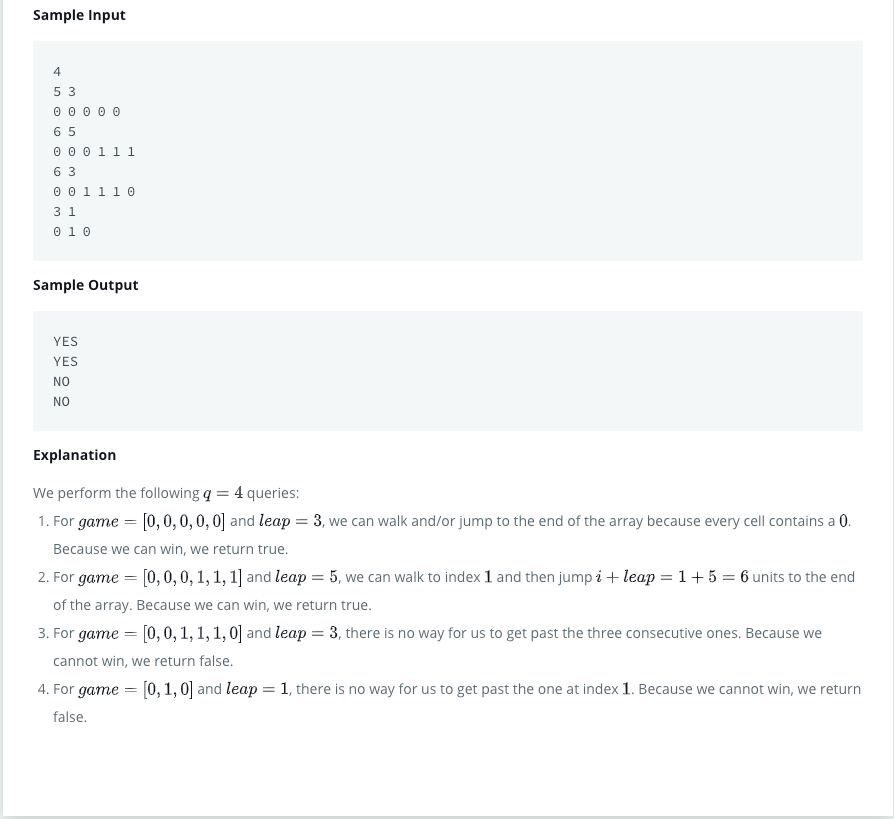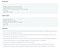# 09/22/2020Photo by Scott Elkins on Unsplash
1. The solutions are coded by myself, and open source just for study.

## Java 1D Array (Part 2)Java 1D Array (Part 2), retrieved from https://www.hackerrank.com/challenges/java-1d-array/problem
`import java.util.*;public class Solution {    public static boolean canWin(int leap, int[] game) {        // Return true if you can win the game; otherwise, return false        return solve(leap, game, 0);    }    private static boolean solve(int leap, int[] game, int i){        if (i < 0 || game[i] == 1){            return false;        }        else if (i>= game.length) {            return true;        }        game[i] = 1;        return solve(leap, game, i + leap) || solve(leap, game, i + 1) || solve(leap, game, i-1);    }    public static void main(String[] args) {        Scanner scan = new Scanner(System.in);        int q = scan.nextInt();        while (q-- > 0) {            int n = scan.nextInt();            int leap = scan.nextInt();            int[] game = new int[n];            for (int i = 0; i < n; i++) {                game[i] = scan.nextInt();            }            System.out.println( (canWin(leap, game)) ? "YES" : "NO" );        }        scan.close();    }}`
`import java.util.*;public class Solution {    public static boolean canWin(int leap, int[] game) {        // Return true if you can win the game; otherwise, return false        return solve(leap, game, 0);    }    private static boolean solve(int leap, int[] game, int i){        if (i >= game.length) {            return true;        }        else if (i < 0 || game[i] == 1) {            return false;        }        game[i] = 1;        return solve(leap, game, i + leap) || solve(leap, game, i + 1) || solve(leap, game, i-1);    }    public static void main(String[] args) {        Scanner scan = new Scanner(System.in);        int q = scan.nextInt();        while (q-- > 0) {            int n = scan.nextInt();            int leap = scan.nextInt();            int[] game = new int[n];            for (int i = 0; i < n; i++) {                game[i] = scan.nextInt();            }            System.out.println( (canWin(leap, game)) ? "YES" : "NO" );        }        scan.close();    }}`

# 11/28/2020Photo by Michiel Leunens on Unsplash

## Java Regex2 — Duplicate WordsJava Regex2 — Duplicate Words, retrieved from https://www.hackerrank.com/challenges/duplicate-word/problem
`import java.util.Scanner;import java.util.regex.Matcher;import java.util.regex.Pattern;public class DuplicateWords {    public static void main(String[] args) {        String regex = "\\b(\\w+)(?:\\W+\\1\\b)+";;        Pattern p = Pattern.compile(regex, Pattern.CASE_INSENSITIVE);        Scanner in = new Scanner(System.in);        int numSentences = Integer.parseInt(in.nextLine());        while (numSentences-- > 0) {            String input = in.nextLine();            Matcher m = p.matcher(input);            // Check for subsequences of input that match the compiled pattern            while (m.find()) {                input = input.replaceAll(m.group(), m.group(1));            }            // Prints the modified sentence.            System.out.println(input);        }        in.close();    }}`

## Conclusion:

I’m not doing so well in these problems. I know, I’ve been occupied by all kinds of stuff recently, but it should not be an excuse. Hope I can keep making progress like I used to (and I have to do that, in fact).

Undergraduate student / Research assistant/ Always curious / Opinions are mine